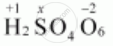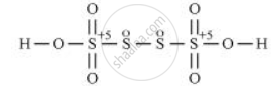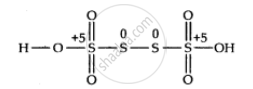Share

# What Are the Oxidation Numbers of the Underlined Elements in Each of the Following and How Do You Rationalise Your Results? H2s4o6 - Chemistry

#### Question

What are the oxidation numbers of the underlined elements in each of the following and how do you rationalise your results?

H2S4O6

#### Solution 1

H2S4O6Now 2(+1) + 4(x) + 6(-2) = 0

=> 2 + 4x - 12 = 0

=> 4x = 10

=> x = +2 1/2

However, O.N. cannot be fractional. Hence, S must be present in different oxidation states in the molecule.The O.N. of two of the four S atoms is +5 and the O.N. of the other two S atoms is 0.

#### Solution 2

By conventional method O.N of S in H_2S_4O_6 =or 2(+1) + 4x + 6(-2) = 0 or x = 2.5(wrong)

But it is wrong because all the four S atoms cannot be in same oxidation state.

By chemical bonding method. The structure of H_2S_4O_6 is shown belowThe O.N. of each of the S-atoms linked with each other in the middle is zero while that of each of the reamining two S-atoms is +5

Is there an error in this question or solution?